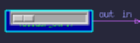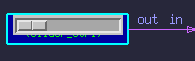### User Slider-Control Gadget:```  This gadget places slider-control on your diagrams.  The slider
becomes active during simulations.  When the slider is moved,
To place a slider in a diagram, instantiate a model that
contains the following function call:

user_slider( label, x, y, your_function, id, min, max );

The label is a character string containing a label for the slider.

The x and y values are the floating-point coordinate
offsets where you want to place the slider on your diagram relative
to the box on which it is instantiated.  An x-y value of (0.0,0.0)
will place the slider at the upper left corner of the box where it is
instantiated.

The your_function is the name of the function that you want
to be called when the slider is moved.  You should define this
function within a DEFINE_GLOBAL: area.

The id is an arbitrary data item that you can use to identify
which of several sliders the entry is coming from.

The min and max values are the integer range of values
to be returned form the slider.

For example:

DEFINE_GLOBAL:

/* Prototype the standard function. */
void user_slider( char *label, float x, float y, void *u_func,
int data_arg, int vmin, int vmax );

/* Define your (user) function. */
void user_slidertest( Widget w, caddr_t client_data,
XmScaleCallbackStruct *call_data )
{
printf("Slider Reading = %d\n", call_data->value );
}

END_DEFINE_GLOBAL.

/* Define your box-model that contains a text-entry form. */
DEFINE_DEVICE_TYPE:  Slider_box

{
user_slider( "Speed", 0.1, 0.1, user_slidertest, 80, 10, 64 );
}

END_DEFINE_DEVICE_TYPE.

To try this example, you would create a diagram that instantiates
a box of type Slider_Box.  If you built and ran the simulation
containing this example-model, you will see a slider appear
on the box.

If you move the slider, it will call the function user_slidertest
which will print, for example:

You can establish any number of sliders and they can call any number
of functions that you define.

Your user functions can do anything they wish with the returned values
from the sliders, such as setting live simulation parameters.

```

### General Purpose Slider Box Model -

As a convenience, there is a general purpose pre-built slider model in the user contributed models library. The model has one slider and one output port. Whenever the slider is moved, it outputs the new value on its output link. You can instantiate any number of these slider-boxes in your diagrams.To use, simply import:
\$CSIM_MODEL_LIBS/user_contributed/generic_slider.sim
Then instantiate boxes of type:   slider_ctrl

Output: Integer

Attributes:
min - Integer, sets a minimum value.
max - Integer, sets a maximum value.
init - Integer, sets the initial slider value.
scale - Float, sets a divisor to scale the integer range (min:max).

There is a complete example in the user_contributed models directory, called generic_slider_example.sim. It instantiates two sliders with test boxes. Moving the slider lights the test box it is connected to and prints the value to your text window. Try it by building and running it.